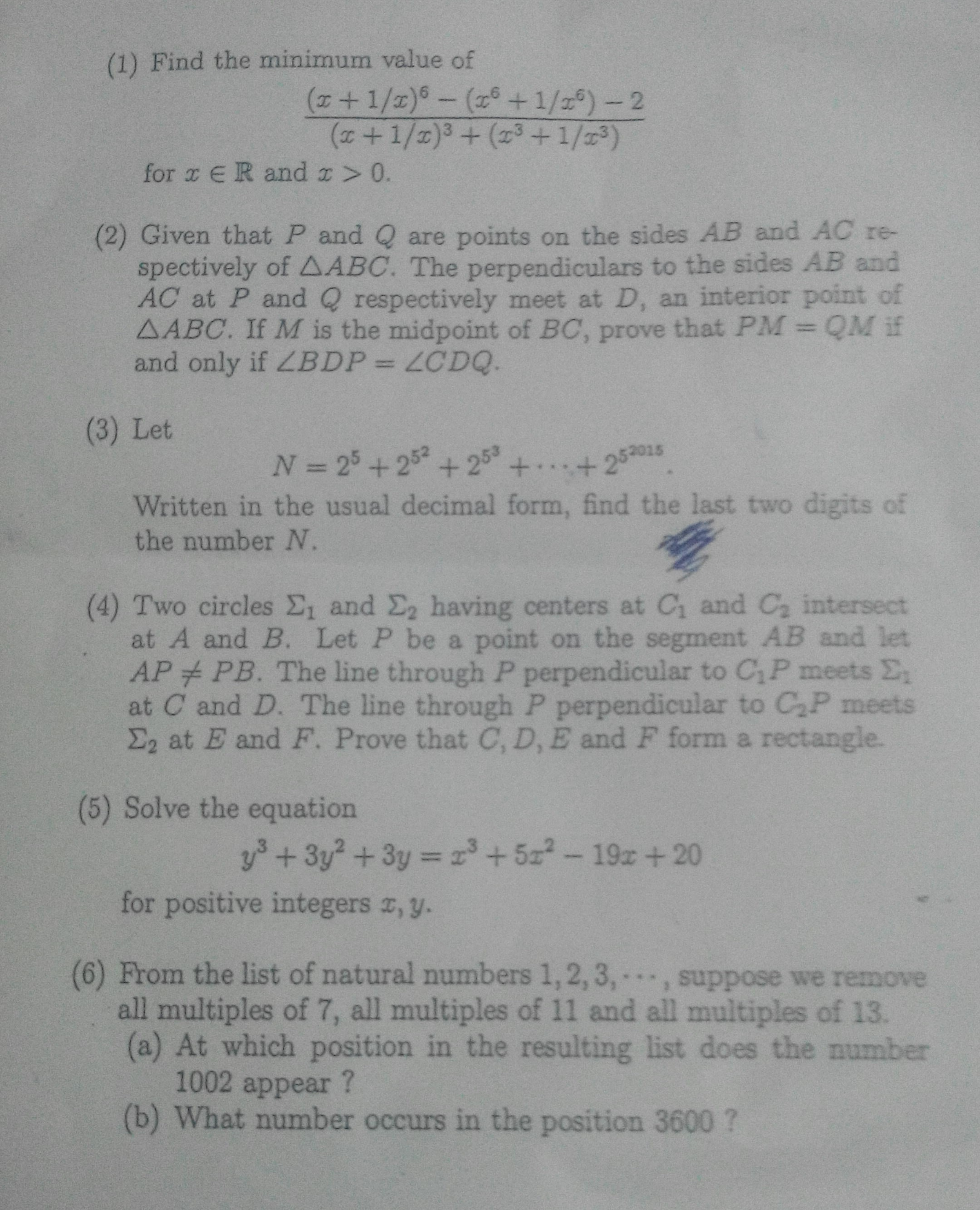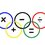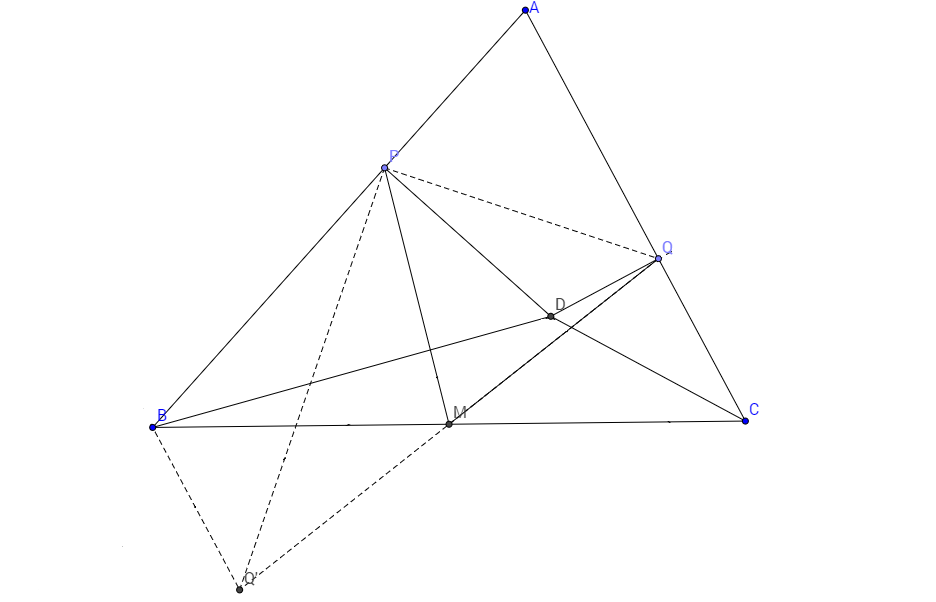# This note has been used to help create the RMO Math Contest Preparation wiki

At last, nervousness comes down. Exam got over. Thanks to Brilliant, it suited my learning style. I like to learn new stuff through discussion and solving new problems,

Now, here is the paper. I think I have done a decent jobNote by Ganesh Ayyappan
5 years, 7 months ago

This discussion board is a place to discuss our Daily Challenges and the math and science related to those challenges. Explanations are more than just a solution — they should explain the steps and thinking strategies that you used to obtain the solution. Comments should further the discussion of math and science.

When posting on Brilliant:

• Use the emojis to react to an explanation, whether you're congratulating a job well done , or just really confused .
• Ask specific questions about the challenge or the steps in somebody's explanation. Well-posed questions can add a lot to the discussion, but posting "I don't understand!" doesn't help anyone.
• Try to contribute something new to the discussion, whether it is an extension, generalization or other idea related to the challenge.

MarkdownAppears as
*italics* or _italics_ italics
**bold** or __bold__ bold
- bulleted- list
• bulleted
• list
1. numbered2. list
1. numbered
2. list
Note: you must add a full line of space before and after lists for them to show up correctly
paragraph 1paragraph 2

paragraph 1

paragraph 2

[example link](https://brilliant.org)example link
> This is a quote
This is a quote
    # I indented these lines
# 4 spaces, and now they show
# up as a code block.

print "hello world"
# I indented these lines
# 4 spaces, and now they show
# up as a code block.

print "hello world"
MathAppears as
Remember to wrap math in $$ ... $$ or $ ... $ to ensure proper formatting.
2 \times 3 $2 \times 3$
2^{34} $2^{34}$
a_{i-1} $a_{i-1}$
\frac{2}{3} $\frac{2}{3}$
\sqrt{2} $\sqrt{2}$
\sum_{i=1}^3 $\sum_{i=1}^3$
\sin \theta $\sin \theta$
\boxed{123} $\boxed{123}$

Sort by:

Let $\boxed{(x+\frac{1}{x})=m}.......(1)$ . Therefore $(x^{3}+\frac{1}{x^{3}})+3(x+\frac{1}{x})=m^{3}$ $\boxed{(x^{3}+\frac{1}{x^{3}})=m^{3}-3m}.......(2)$ Squaring both sides , we get $\boxed{(x^{6}+\frac{1}{x^{6}})=m^{6}+9m^{2}-6m^{4}-2}........(3)$ Now rewriting the given expression in terms of $m$ we get $=\frac{m^{6}-(m^{6}+9m^{2}-6m^{4}-2)-2}{m^{3}+m^{3}-3m}$ $=\frac{6m^{4}-9m^{2}}{2m^{3}-3m}$ $=3m$ By $A.M-G.M$ $x+\frac{1}{x}\geq2$ Therefore $3m\geq6$ Hence the minimum value of the given expression is $6$

- 5 years, 7 months ago

Did the same

- 5 years, 7 months ago

Question 5 is trivial. Add 1 to the LHS and then it becomes a perfect cube. Then try proving that the RHS is a cube lying between x^3 and (x+2)^3 forcing it to be (x+1)^3. thus u obtain that x=y. Substitute that and get solutions as (1,1),(10,10)

- 5 years, 7 months ago

Perfect solution!

- 5 years, 7 months ago

$\boxed{CP=CD,EP=PF}.....(1)$ (Since $C_{1}P,C_{2}P$ are perpendicular to $CD,EF$ respectively) Angle$CPF$ =Angle$EPF$ Angle$CPE$ =Angle$FPD.....(2)$ Using $(1),(2)$ Triangle CPF is congruent to triangle EPD (BY SAS Test)

Triangle CPE is congruent to triangle FPD (BY SAS Test)

Therefore CF=ED,CE=FD...(I). And Angle$FCD$ =Angle$EDC$ Which implies CF||ED....(3) Similarly we prove CE||FD...(4) Hence using (I),(3),(4) we prove that CEDF is a rectangle.

- 5 years, 7 months ago

What is the cutoff for qualifying to the next round?

- 5 years, 7 months ago

I too want to know the same .... i am nervous about that .. If anyone gets to know it ... PLEASE post it in this discussion ... let the Tamil Nadu people in Brilliant know it ...

- 5 years, 7 months ago

is trichy a centre for RMO

- 5 years, 6 months ago

Bro ... I dont know abt trichy ...

- 5 years, 6 months ago

i hope pic is clear ... else jus reply to me ... i will type out the full question

- 5 years, 7 months ago

3) 80

- 5 years, 7 months ago

Yeah .. I too got the Same

- 5 years, 7 months ago

What method did u use ? mod 100 ?

- 5 years, 7 months ago

That is probably the easiest method.

- 5 years, 7 months ago

But can u explain how did u solve that question by mod 100 ? I could not solve it that way

- 5 years, 7 months ago

$32^{5}=2^{25} \equiv 2^{10} \times 2^{10} \times 2^{5} \equiv 24^{2} \times 32 \equiv 32 \pmod{100}$. Hence last two digits of every fifth power of $32$ is $32$. Hence last two digits of $N$ is last two digits of $2015 \times 32=64480$ which is $80$.

- 5 years, 7 months ago

Is 6(a) 721 and 6(b) 5004?

- 5 years, 7 months ago

Yeah ... I too got the same

- 5 years, 7 months ago

Yeah .I too got the same.

- 5 years, 7 months ago

hey mohan i got 5005 dah

- 5 years, 7 months ago

People .. Any idea abt cutoff?? ..

- 5 years, 7 months ago

Q3) Clearly $N \equiv 0 \equiv 80 \quad (\mod 4)$.

Now we construct a table for $2^{5^{n}} \quad (\mod 25)$.

$\Large{\underline { \begin{matrix} n & & n\quad \left( \mod 25 \right) \end{matrix} } \\ \begin{matrix} 1 & & 7 \\ 2 & & 7 \\ 3 & & 7 \\ & . & \\ & . & \\ & . & \\ & & \end{matrix}}$

This gives $N \equiv 5 \equiv 80 \quad (\mod 25)$. Hence the answer is $\boxed{80}$

- 5 years, 7 months ago

Both your and Svatejas's solutions are good.

What about question 5? How you did?

- 5 years, 7 months ago

I did it like Shrihari B.

- 5 years, 7 months ago

Ok. Which book do you have regarding Olympiad geometry?

- 5 years, 7 months ago

In mathematics for me, Brilliant is enough and some other sites, which i believe you follow (Ao...)

- 5 years, 7 months ago

What do you guys expect the cutoff to be? I'm really nervous about the result as this is my first time writing RMO.

- 5 years, 7 months ago

Mine too, I think the cut off would be 51

- 5 years, 7 months ago

Ha that makes me kind of more nervous since I'm expcecting about 45-55. :)

- 5 years, 7 months ago

Me I am getting only 17-22.

- 5 years, 7 months ago

Ya, AOPS is full of resources regarding any topic, contests.

Also, Do you appeared in RMO? From Delhi region?

- 5 years, 7 months ago

I got #1 and #3 right. For #4 I showed that CDEF is a parallelogram since P is the midpoint of CD and EF. Finally for #6a) I got 722 by some arithmetic an error. How much marks would I be approximately getting? Any guesses for the cutoff? Thanks!

- 5 years, 7 months ago

Friends ... The RMO results are out ... Fortunately I hav cleared it and now can write INMO ... but i feel nervous coz i am hardly able to do 1 sum frm previous year papers ... so i wish to get some tips regareding INMO

- 5 years, 6 months ago

Congratulations!

- 5 years, 6 months ago

thanks ... wat about you brother? .. i tink ur region results came before ours ...

- 5 years, 6 months ago

I have got the proof for the 4 th one

- 5 years, 5 months ago

@ Ganesh Ayyappan Hi I am Anurag from Mumbai and currently, I am in 8th standard and selected for RMO.I am able to solve only three or four problems from each RMO paper or even 2 if it is difficult. For sums I am not able to solve, I am able to get through halfway and then I can't solve because I don't the formula or else, I am missing something. Is 3 or 4 problems from 6 alright to get through RMO and go to INMO? Please let me know. Do follow me. I will be readily answering any sum on Brilliant.

- 3 years, 10 months ago

LOL you were damn lucky to get selected.

- 2 years, 10 months ago

1) 6

3) 80

5) x=y=1 & x=y=10

6) A - 721 & B - 5004

i verified once that all are right .. and i tink some of my friends havent noticed that this discussion is put up ....

@Harsh Shrivastava @Adarsh Kumar @Mehul Chaturvedi @Kartik Sharma and some more of my BRILLIANT friends ... awaiting ur valuable replies

Also ... can sum1 post soln of Q2 & 4??

- 5 years, 7 months ago

What do you expect the cutoff to be?

- 5 years, 7 months ago

Since i do not hav any idea .. Im asking here ...

- 5 years, 7 months agoDiagram : Solution to problem 2. @Ganesh Ayyappan Here is a solution for the problem (2).

$Sol^{n}$:

$1st$ case: When $\angle BDP = \angle CDQ$ then $MP = MQ.$

Constructions: Produce $QM$ to $Q'$ such that $MQ = MQ'.$ Join $BQ' , PQ$ and $PQ'.$

Here clearly, quadrilateral $BQCQ'$ is a $|| gm$. $=>$ $\angle BQ' = CQ$ --- $$ and also, $\angle Q'BM = \angle C$ $=>$ $\angle Q'BP = \angle B +\angle C = 180^{\circ}- \angle A = \angle PDQ$ --- $$

In $\triangle BDP$ and $\Delta CDQ,$ $\angle BDP = \angle CDQ$ and $\angle BPD = \angle CQD$ $=90^{\circ}.$ $=>$ $\Delta BDP \sim \Delta CDQ$ $=>$ $\dfrac{BP}{CQ}= \dfrac{DP}{DQ}$ $=>$ $\dfrac{BP}{BQ'} = \dfrac{DP}{DQ}$ (Using - $$ ) $=>$ $\dfrac{BP}{DP} = \dfrac{BQ'}{DQ}$

Now, in $\Delta BQ'P$ & $\Delta PDQ$ , $\dfrac{BP}{DP} = \dfrac{BQ'}{DQ}$ and $\angle Q'BP = \angle PDQ$ [From $eq^{n}$] - $2$ $=>$ $\Delta BQ'P \sim \Delta PDQ$ $=>$ $\angle BPQ' = \angle DPQ$ $=>$ $\angle BPQ' + \angle Q'PQ = \angle Q'PQ + \angle DPQ$ $=>$ $90^{\circ} = \angle Q'PQ + \angle DPQ = \angle QPQ'$ $=>$ $\Delta QPQ'$ is right angled at $P$.

And we know that the midpoint of the hypotenuse of a right angled triangle is circumcenter of that $\Delta$. $=>$ $MQ = MP$ $= MQ'$

Now, just reverse this process to prove that: If $MP = MQ$ then $\angle BDP = \angle CDQ$.

- 4 years, 2 months ago

Problem 4 is easy. One can easily notice that $EP = FP$ and $DP = CP$ [Because, the $\perp$ from the center to the chord bisects the chord]. => $FDEC$ is a $|| gm.$ Now, since $DACB$ is cyclic, $DP\times CP = AP\times BP = DP^{2}$ -- $1$. Similarly, since $FAEB$ is cyclic, $EP\times FP = AP\times BP = FP^{2}$-- $2$ The two results $1 and 2$ tells that, $DP = FP => 2DP = 2FP => DC = FE$. So, quad $DFCE$ is a $||gm$ with the diagonals equal. => $CEDF$ is a rectangle

- 4 years, 2 months ago

I think this region's paper was the easiest one.

- 5 years, 7 months ago

In fact i found this the toughest paper !

- 5 years, 6 months ago

@Shrihari B I feel that Mumbai region was the hardest. Definitely my region was the easiest but still I couldn't quality that :( Why aren't you participating in the INMO board anyone?

- 5 years, 6 months ago

dont worry bro ... u r a 9th grader i guess .. u still hav 2 yrs ... for me .. this is the first and last time i cud hav written RMO (I was eligible to write RMO during 9th and 10th but wasnt aware about what was it) ... so only i am nervous about INMO ...

besides being a 14 yr old ... u are a level 5 solver in all topics u hav took ... tats really appreciable ... so dont worry abt this yr RMO .... GOOD LUCK

- 5 years, 6 months ago

I am actually in 8th

- 5 years, 6 months ago

oh ... well and good .... u hav 3 more yrs .. GOOD LUCK ... dont at all regret or tink abt wat u did this RMO ... i am telling this coz i am able to see ur potential through the novel methods u suggest and a LEVEL 5 guy in Algebra Geometry and Combinatorics .... in 8th ... i wasnt this well-equipped bro ... u hav a long way to go ... Wish u a BRIGHT COLORFUL FUTURE in the field u want to take

- 5 years, 6 months ago

Thank you very much for your encouragement. Wish all the very best to everyone appearing for INMO.

- 5 years, 6 months ago

I actually became level 5 by mostly doing level 1-3 problems, especially the unrated problems worth 100 points.

- 5 years, 6 months ago

its okay ... do level 4 frm now onwards ... do level 5 ocassionally ... make sure u do gud progress in algebra geometry and inequalities

- 5 years, 6 months ago

Hi Svatejas sorry for not being able to participate in the INMO practise board.Actually I had my INMO training camp for four days and so hardly got any time to log in to brilliant. But it gets over tomorrow so i will be participating

- 5 years, 6 months ago

No problem. I was just asking.

- 5 years, 6 months ago

Someone please post solution for q6, it was the easiest but sill want to know how others did it

- 5 years, 6 months ago

Use Principle of Inclusion and Exclusion.

- 5 years, 6 months ago

Got through with part a, finding part b difficult

- 5 years, 6 months ago